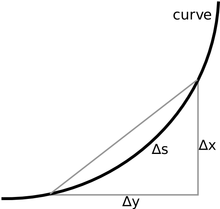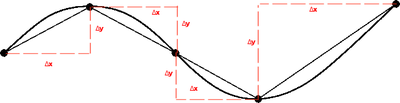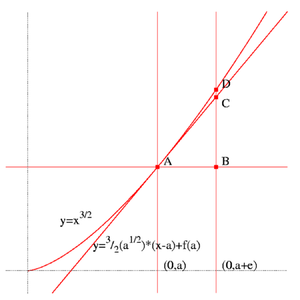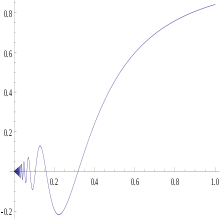# Arc length

﻿
Arc length

Determining the length of an irregular arc segment is also called rectification of a curve. Historically, many methods were used for specific curves. The advent of infinitesimal calculus led to a general formula that provides closed-form solutions in some cases.

## General approachApproximation by multiple linear segments

A curve in the plane can be approximated by connecting a finite number of points on the curve using line segments to create a polygonal path. Since it is straightforward to calculate the length of each linear segment (using the Pythagorean theorem in Euclidean space, for example), the total length of the approximation can be found by summing the lengths of each linear segment.

If the curve is not already a polygonal path, better approximations to the curve can be obtained by following the shape of the curve increasingly more closely. The approach is to use an increasingly larger number of segments of smaller lengths. The lengths of the successive approximations do not decrease and will eventually keep increasing—possibly indefinitely, but for smooth curves this will tend to a limit as the lengths of the segments get arbitrarily small.

For some curves there is a smallest number L that is an upper bound on the length of any polygonal approximation. If such a number exists, then the curve is said to be rectifiable and the curve is defined to have arc length L.

## Definition

Let C be a curve in Euclidean (or, more generally, a metric) space X = Rn, so C is the image of a continuous function f : [a, b] → X of the interval [a, b] into X.

From a partition a = t0 < t1 < ... < tn−1 < tn = b of the interval [a, b] we obtain a finite collection of points f(t0), f(t1), ..., f(tn−1), f(tn) on the curve C. Denote the distance from f(ti) to f(ti+1) by d(f(ti), f(ti+1)), which is the length of the line segment connecting the two points.

The arc length L of C is then defined to be$L(C) = \sup_{a=t_0 < t_1 < \cdots < t_n = b} \sum_{i = 0}^{n - 1} d(f(t_i), f(t_{i+1}))$

where the supremum is taken over all possible partitions of [ab] and n is unbounded.

The arc length L is either finite or infinite. If L < ∞ then we say that C is rectifiable, and is non-rectifiable otherwise. This definition of arc length does not require that C is defined by a differentiable function. In fact in general, the notion of differentiability is not defined on a metric space.

A curve may be parameterized in many ways. Suppose C also has the parameterization g : [cd] → X. Provided that f and g are injective, there is a continuous monotone function S from [ab] to [cd] so that g(S(t)) = f(t) and an inverse function S−1 from [cd] to [ab]. It is clear that any sum of the form$\sum_{i = 0}^{n - 1} d(f(t_i), f(t_{i+1}))$ can be made equal to a sum of the form$\sum_{i = 0}^{n - 1} d(g(u_i), g(u_{i+1}))$ by taking ui = S(ti), and similarly a sum involving g can be made equal to a sum involving f. So the arc length is an intrinsic property of the curve, meaning that it does not depend on the choice of parameterization.

The definition of arc length for the curve is analogous to the definition of the total variation of a real-valued function.

## Finding arc lengths by integrating

Consider a real function f(x) such that f(x) and$f'(x)=\frac{dy}{dx}$ (its derivative with respect to x) are continuous on [ab]. The length s of the part of the graph of f between x = a and x = b can be found as follows:

Consider an infinitesimal part of the curve ds (or consider this as a limit in which the change in s approaches ds). According to Pythagoras' theorem ds2 = dx2 + dy2, from which:

ds2 = dx2 + dy2$\frac{ds^2}{dx^2}=1+\frac{dy^2}{dx^2}$$\frac{ds^2}{dx^2}=1+\left(\frac{dy}{dx}\right)^2$$\frac{ds}{dx}=\sqrt{1+\left(\frac{dy}{dx}\right)^2}$$ds=\sqrt{1+\left(\frac{dy}{dx}\right)^2}dx$$s = \int_{a}^{b} \sqrt { 1 + [f'(x)]^2 }\, dx.$

If a curve is defined parametrically by x = X(t) and y = Y(t), then its arc length between t = a and t = b is$s = \int_{a}^{b} \sqrt { [X'(t)]^2 + [Y'(t)]^2 }\, dt.$

This is more clearly a consequence of the distance formula where instead of a Δx and Δy , we take the limit. A useful mnemonic is$s = \lim \sum_a^b \sqrt { \Delta x^2 + \Delta y^2 } = \int_{a}^{b} \sqrt { dx^2 + dy^2 } = \int_{a}^{b} \sqrt { \left(\frac{dx}{dt}\right)^2 + \left(\frac{dy}{dt}\right)^2 }\,dt.$

If a function is defined in polar coordinates by r = f(θ) then the arc length is given by$s = \int_a^b \sqrt{r^2+\left(\frac{dr}{d\theta}\right)^2} \, d\theta.$

In most cases, including even simple curves, there are no closed-form solutions of arc length and numerical integration is necessary.

Curves with closed-form solution for arc length include the catenary, circle, cycloid, logarithmic spiral, parabola, semicubical parabola and (mathematically, a curve) straight line. The lack of closed form solution for the arc length of an elliptic arc led to the development of the elliptic integrals.

### DerivationFor a small piece of curve, ∆s can be approximated with the Pythagorean theoremA representative linear element of the function y=t 5, x = t 3

In order to approximate the arc length of the curve, it is split into many linear segments. To make the value exact, and not an approximation, infinitely many linear elements are needed. This means that each element is infinitely small. This fact manifests itself later on when an integral is used.

Begin by looking at a representative linear segment (see image) and observe that its length (element of the arc length) will be the differential ds. We will call the horizontal element of this distance dx, and the vertical element dy.

The Pythagorean theorem tells us that$ds = \sqrt{dx^2 + dy^2}.\,$

Since the function is defined in time, segments (ds) are added up across infinitesimally small intervals of time (dt) yielding the integral$\int_a^b \sqrt{\bigg(\frac{dx}{dt}\bigg)^2+\bigg(\frac{dy}{dt}\bigg)^2}\,dt,$

If y is a function of x, so that we could take t = x, then we have:$\int_a^b \sqrt{1+\bigg(\frac{dy}{dx}\bigg)^2}\,dx,$

which is the arc length from x = a to x = b of the graph of the function ƒ.

For example, the curve in this figure is defined by$\begin{cases} y = t^5, \\ x = t^3. \end{cases}$

Subsequently, the arc length integral for values of t from -1 to 1 is$\int_{-1}^1 \sqrt{(3t^2)^2 + (5t^4)^2}\,dt = \int_{-1}^1 \sqrt{9t^4 + 25t^8}\,dt.$

Using computational approximations, we can obtain a very accurate (but still approximate) arc length of 2.905. An expression in terms of the hypergeometric function can be obtained: it is$\textstyle 2\,{}_2F_1\left(-\frac{1}{2},\frac{3}{4};\frac{7}{4};-\frac{25}{9}\right).$

### Another way to obtain the integral formulaApproximation by multiple hypotenuses

Suppose that there exists a rectifiable curve given by a function f(x). To approximate the arc length S along f between two points a and b in that curve, construct a series of right triangles whose concatenated hypotenuses "cover" the arc of curve chosen as shown in the figure. For convenience, the bases of all those triangles can be set equal to Δx, so that for each one an associated Δy exists. The length of any given hypotenuse is given by the Pythagorean Theorem:$\sqrt {\Delta x^2 + \Delta y^2}$

The summation of the lengths of the n hypotenuses approximates S:$S \sim \sum_{i=1}^n \sqrt { \Delta x_i^2 + \Delta y_i^2 }$

Multiplying the radicand by$\frac{\Delta x^2}{\Delta x^2}$ produces:$\sqrt { \Delta x^2 + \Delta y^2 }=\sqrt{ ({\Delta x^2 + \Delta y^2})\,\frac{\Delta x^2}{\Delta x^2}}=\sqrt { 1 + \frac{\Delta y^2}{\Delta x^2}}\,\Delta x=\sqrt { 1 + \left(\frac{\Delta y} {\Delta x} \right)^2 }\,\Delta x$

Then, our previous result becomes:$S \sim \sum_{i=1}^n \sqrt { 1 + \left(\frac{\Delta y_i} {\Delta x_i} \right)^2 }\,\Delta x_i$

As the length Δx of these segments decreases, the approximation improves. The limit of the approximation, as Δx goes to zero, is equal to S:$S = \lim_{\Delta x_i \to 0} \sum_{i=1}^\infty \sqrt { 1 + \left(\frac{\Delta y_i}{\Delta x_i} \right)^2 }\,\Delta x_i = \int_{a}^{b} \sqrt { 1 + \left(\frac{dy}{dx}\right)^2 } \,dx = \int_{a}^{b} \sqrt{1 + \left [ f' \left ( x \right ) \right ] ^2} \, dx.$

### Another proof

We know that the formula for a line integral is$\int_a^b f(x,y) \sqrt{x'[t]^2+y'[t]^2} \, dt$. If we set the surface f(x,y) to 1, we will get arc length multiplied by 1, or$\int_a^b \sqrt{x'[t]^2+y'[t]^2} dt$. If x = t, and y = f(t), then y = f(x), from when x is a to when x is b. If we set these equations into our formula we get:$\int_a^b \sqrt{1+f'(x)^2} \, dx$ (Note: If x = t then dt = dx). This is the arc length formula.

### Arcs of circles

The length of an arc of a circle is the central angle divided by 360° multiplied by the circumference.

The circumference of a circle is C = 2πr, where r is the radius, or C = πd, where d is the diameter.

Arc lengths are denoted by s, since arcs "subtend" an angle.

If the angle measurement is in radians then s = rθ where r is the radius and θ is the subtended angle. The units of s will be the same as that of the radius.

In a semicircle, s = πr.

## Historical methods

### Ancient

For much of the history of mathematics, even the greatest thinkers considered it impossible to compute the length of an irregular arc. Although Archimedes had pioneered a way of finding the area beneath a curve with his method of exhaustion, few believed it was even possible for curves to have definite lengths, as do straight lines. The first ground was broken in this field, as it often has been in calculus, by approximation. People began to inscribe polygons within the curves and compute the length of the sides for a somewhat accurate measurement of the length. By using more segments, and by decreasing the length of each segment, they were able to obtain a more and more accurate approximation. In particular, by inscribing a polygon of many sides in a circle, they were able to find approximate values of π.

### 1600s

In the 17th century, the method of exhaustion led to the rectification by geometrical methods of several transcendental curves: the logarithmic spiral by Evangelista Torricelli in 1645 (some sources say John Wallis in the 1650s), the cycloid by Christopher Wren in 1658, and the catenary by Gottfried Leibniz in 1691.

In 1659, Wallis credited William Neile's discovery of the first rectification of a nontrivial algebraic curve, the semicubical parabola.

### Integral form

Before the full formal development of the calculus, the basis for the modern integral form for arc length was independently discovered by Hendrik van Heuraet and Pierre de Fermat.

In 1659 van Heuraet published a construction showing that arc length could be interpreted as the area under a curve—this integral, in effect—and applied it to the parabola. In 1660, Fermat published a more general theory containing the same result in his De linearum curvarum cum lineis rectis comparatione dissertatio geometrica.Fermat's method of determining arc length

Building on his previous work with tangents, Fermat used the curve$y = x^{3/2} \,$

whose tangent at x = a had a slope of$\textstyle {3 \over 2} a^{1/2}$

so the tangent line would have the equation$y = \textstyle {3 \over 2} {a^{1/2}}(x - a) + f(a).$

Next, he increased a by a small amount to a + ε, making segment AC a relatively good approximation for the length of the curve from A to D. To find the length of the segment AC, he used the Pythagorean theorem:\begin{align} AC^2 &{}= AB^2 + BC^2 \\ &{} = \textstyle \varepsilon^2 + {9 \over 4} a \varepsilon^2 \\ &{}= \textstyle \varepsilon^2 \left (1 + {9 \over 4} a \right ) \end{align}

which, when solved, yields$AC = \textstyle \varepsilon \sqrt { 1 + {9 \over 4} a\ }.$

In order to approximate the length, Fermat would sum up a sequence of short segments.

## Curves with infinite lengthThe Koch curve.The graph of xsin(1/x).

As mentioned above, some curves are non-rectifiable, that is, there is no upper bound on the lengths of polygonal approximations; the length can be made arbitrarily large. Informally, such curves are said to have infinite length. There are continuous curves on which every arc (other than a single-point arc) has infinite length. An example of such a curve is the Koch curve. Another example of a curve with infinite length is the graph of the function defined by f(x) = x sin(1/x) for any open set with 0 as one of its delimiters and f(0) = 0. Sometimes the Hausdorff dimension and Hausdorff measure are used to "measure" the size of infinite-length curves.

## Generalization to (pseudo-)Riemannian manifolds

Let M be a (pseudo-)Riemannian manifold, γ : [0, 1] → M a curve in M and g the (pseudo-) metric tensor.

The length of γ is defined to be$\ell(\gamma)=\int_{0}^{1} \sqrt{ \pm g(\gamma'(t),\gamma '(t)) } \, dt,$

where γ'(t)Tγ(t)M is the tangent vector of γ at t. The sign in the square root is chosen once for a given curve, to ensure that the square root is a real number. The positive sign is chosen for spacelike curves; in a pseudo-Riemannian manifold, the negative sign may be chosen for timelike curves.

In theory of relativity, arc-length of timelike curves (world lines) is the proper time elapsed along the world line.

Wikimedia Foundation. 2010.

### Look at other dictionaries:

• Arc welding — uses a welding power supply to create an electric arc between an electrode and the base material to melt the metals at the welding point. They can use either direct (DC) or alternating (AC) current, and consumable or non consumable electrodes.… …   Wikipedia

• Length (disambiguation) — Length in its basic meaning is the long dimension of an object.Length may also refer to: *Length (phonetics), in phonetics **Vowel length **Geminate consonant *Arc length *Length of a module, in abstract algebra *Length of a polynomial *Vector… …   Wikipedia

• Arc (geometry) — A circular sector is shaded in green. Its curved boundary of length L is a circular arc. In geometry, an arc is a closed segment of a differentiable curve in the two dimensional plane; for example, a circular arc is a segment of the circumference …   Wikipedia

• Arc (album) — Infobox Album | Name = Arc Type = Live album Artist = Neil Young Released = 1991 Recorded = 1991 North American tour Genre = Noise rock Computer music Electronic music Length = 35:00 Label = Reprise/Warner Bros. Records 26769 Producer = Neil… …   Wikipedia

• Arc (Savoie) — Infobox River | river name = Arc caption = origin = Graian Alps mouth = Isère coord|45|33|46|N|6|12|13|E|name=Isère Arc|display=inline,title basin countries = France length = 128 km elevation = 2760 m discharge = watershed = The Arc is a 128 km… …   Wikipedia

• Arc (Provence) — Infobox River | river name = Arc image size = 200px caption = The Arc in Roquefavour origin = near Pourcieux mouth = Étang de Berre coord|43|30|24|N|5|6|49|E|name=Étang de Berre Arc|display=inline,title basin countries = France length = 83 km… …   Wikipedia

• Arc of a Diver — Infobox Album | Name = Arc of a Diver Type =Album Artist = Steve Winwood Released = 31 December 1980 Recorded = Genre = Rock, Blue eyed soul Length = 40:02 Label = Island Records Producer = Steve Winwood Reviews = *Allmusic Rating|4.5|5… …   Wikipedia

• Arc'tan'gent — Infobox Album Name = arc tan gent Type = studio Artist = earthtone9 Released = September 25 2000 Recorded = 2000, Philia Studios, Henley on Thames; Backstage, Ripley Genre = Progressive metal, alternative metal Length = Label = Copro COP017… …   Wikipedia

• Arc of the Testimony — Infobox Album Name = Arc of the Testimony Type = studio Artist = Arcana Released = 1997 Recorded = Genre = Jazz fusion Length = Label = Producer = Reviews = * Allmusic Rating|4.5|5 [http://www.allmusic.com/cg/amg.dll?p=amg sql=10:h9fexqrjldke… …   Wikipedia

• Gas metal arc welding — RMD redirects here. RMD may also refer to IRA Required Minimum Distributions. Gas metal arc welding …   Wikipedia